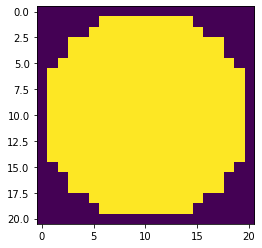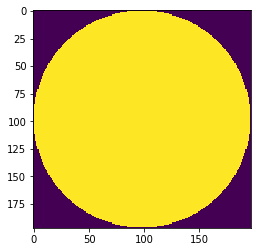GeeksforGeeks App
Open AppBrowser
Continue

# Mahotas – Getting Disk Structuring Element of given radius

In this article we will see how we can get binary disk structuring element of given radius of the image in mahotas. A flat structuring element is a binary valued neighborhood, either 2-D or multidimensional, in which the true pixels are included in the morphological computation, and the false pixels are not. The center pixel of the structuring element, called the origin, identifies the pixel in the image being processed.

In this tutorial we will use “lena” image, below is the command to load it.

`mahotas.demos.load('lena')`

Below is the lena imageIn order to do this we will use `mahotas.disk` method

Argument : It takes integer as argument

Return : It returns image object

Below is the implementation

 `# importing required libraries``import` `mahotas``import` `numpy as np``from` `pylab ``import` `gray, imshow, show``import` `os`` ` `# getting disk of given radius``disk ``=` `mahotas.disk(``10``)`` ` `# showing disk image``imshow(disk)``show()`

Output :Another example

 `# importing required libraries``import` `mahotas``import` `numpy as np``from` `pylab ``import` `gray, imshow, show``import` `os`` ` `# getting disk of given radius``disk ``=` `mahotas.disk(``98``)`` ` `# showing disk image``imshow(disk)``show()`

Output :My Personal Notes arrow_drop_up